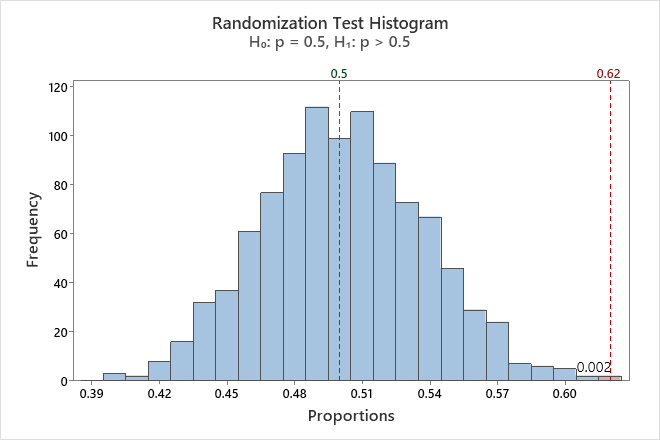# Example of Randomization test for 1-sample proportion

An editor of a video game web site wants to determine whether the proportion of readers that own a PlayStation console is greater than 0.5.

1. Choose Calc > Resampling > Randomization Test for 1-Sample Proportion.
2. From the drop down, select Summarized data.
3. In Number of events, enter 124.
4. In Number of trials, enter 200.
5. In Hypothesized proportion, enter 0.5.
6. Click Options.
7. From Alternative hypothesis, select Proportion > hypothesized value.
8. Enter 1 in Base for random number generator.
###### Note

Using Base for random number generator ensures that your results match the example.

9. Click OK in each dialog box.

## Interpret the results

The alternative hypothesis states that the proportion of readers that own a PlayStation console is greater than 0.5. Because the p-value is 0.002, which is less than the significance level of 0.05, the editor rejects the null hypothesis and concludes that the proportion of readers that own a PlayStation console is greater than 0.5. The histogram shows that the bootstrap distribution appears to be normal, so the editor can trust the results.### Randomization Test Histogram

Observed Sample N Proportion 200 0.620000
Randomization Test Null hypothesis H₀: p = 0.5 Alternative hypothesis H₁: p > 0.5 Number of Resamples Average P-Value 1000 0.49942 0.002
By using this site you agree to the use of cookies for analytics and personalized content.  Read our policy